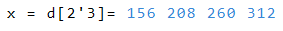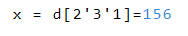Data fields

Description of the calculation with data fields

Work with data fields

This page describes the calculation with multidimensional data fields. For more information of definition and caltulation see the page Creating and Calculating lists and tables.

Basicly

• Two data fields can be operands of a mathematical expression

• The elements of the first array are calculated with the corresponding elements of the second array

• If the array size is different, the extra elements of the longer field are ignored

Example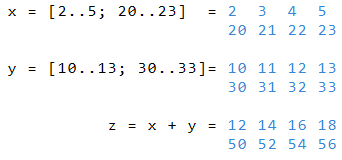In the next example, excessive elements of b are ignored.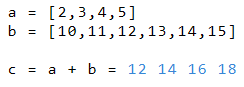In this example, the third line of b is ignored.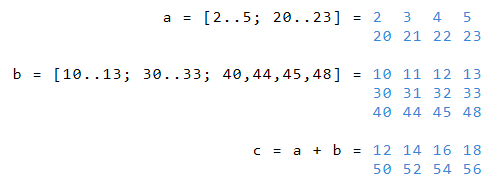This example shows the addition of a to rows 1 and 3 of variable b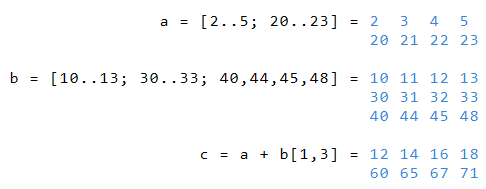Create more-dimensional data arrays

In the above examples, each index of b is calculated with the corresponding index of b. Alternatively, RedCrab can compute arrays in which each index of a is computed with each index of b.

• The result is a multidimensional field of size a * b

• The empty brackets after c determine the result of the following calculation as a multidimensional array.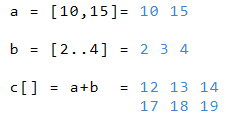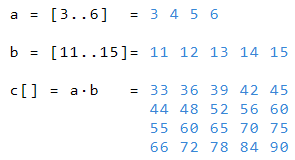The result of multiplying the one-dimensional array a and the two-dimensional array c is the three-dimensional array d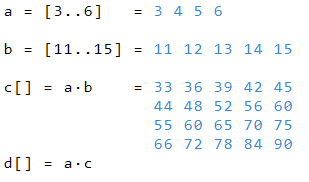• On the array level can be accessed via index

• The example below shows the four levels of the four-dimensional array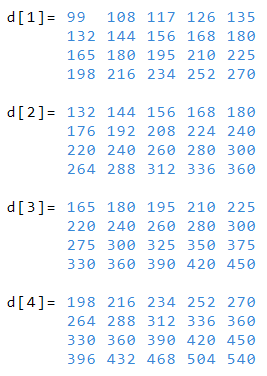• The following example shows how you can read the third column in the second level of d

• The apostrophe is the delimiter